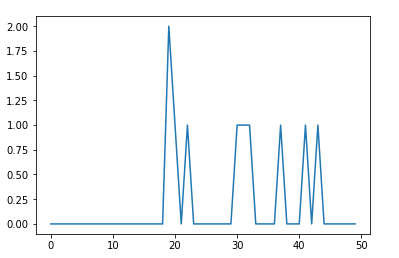﻿ NumPy: Compute the line graph of a set of data - w3resource# NumPy: Compute the line graph of a set of data

## NumPy: Array Object Exercise-117 with Solution

Write a NumPy program to compute the line graph of a set of data.

Sample Solution:

Python Code:

``````import numpy as np
import matplotlib.pyplot as plt
arr = np.random.randint(1, 50, 10)
y, x = np.histogram(arr, bins=np.arange(51))
fig, ax = plt.subplots()
ax.plot(x[:-1], y)
fig.show()
```
```

Sample Output:

``````

Python Code Editor:

Have another way to solve this solution? Contribute your code (and comments) through Disqus.

What is the difficulty level of this exercise?

Test your Python skills with w3resource's quiz

﻿

## Python: Tips of the Day

Set comprehension:

```>>> m = {x ** 2 for x in range(5)}
>>> m
{0, 1, 4, 9, 16}
```